## Excel BINOMDIST function

The BINOMDIST function returns the individual term binominal distribution probability, which can be used to calculate the probability of a specified number of successes out of a given number of trials.#### BINOMDIST function VS. BINOM.DIST function:

Starting from Excel 2010, the BINOMDIST function has been replaced by the BINOM.DIST function. Although they can both be used to calculate the probability of a specified number of successes out of a given number of trials, the BINOM.DIST function may provide better accuracy.

For future uses, the BINOM.DIST function is more recommended, because the BINOMDIST function may not be available in future versions of Excel.

#### Syntax

BINOMDIST(number_s, trials, probability_s, cumulative)

#### Arguments

• Number_s (required): The number of successes in trials;
• Trials (required): The number of independent trials;
• Probability_s (required): The probability of success per trial;
• Cumulative (required): A logical value used to determine the form of the function. It can be:
-- TRUE: use the cumulative distribution function;
-- FLASE: use the probability mass function.

#### Remarks

1. If the arguments “number_s” and “trials” are not integers, they will be truncated;
2. The #NUM! error value occurs when any of the following conditions is met:
-- “Number_s” < 0;
-- “Number_s” > “trials”;
-- “Probability_s” < 0, or “Probability_s” > 1;
3. The #VALUE! error value occurs if any of the supplied “number_s”, “trials” or “probability_s” are non-numeric;
4. Supposing x = number_s, n = trials, and p = probability_s, the equations for the cumulative distribution function and the probability mass function are as follows:
-- The equation for the cumulative distribution function: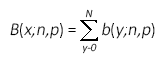-- The equation for the probability mass function: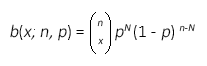where: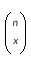is COMBIN(n,x).

#### Return value

It returns a numeric value.

#### Example

In the following table, there are 6 successes in 14 trials, and the probability of success in each trial is 0.5. To calculate the probability of at most 6 successes out of 14 trials and the probability of 6 successes out of 14 trials separately, you also can apply the BINOMDIST function to get it done.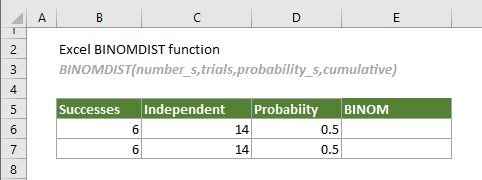Apply the BINOMDIST function to calculate the probability of 6 successes out of 14 trials.

Select a cell (In this case I select E6), copy or enter the formula below and press Enter to get the result.

=BINOMDIST(B6,C6,D6,FALSE)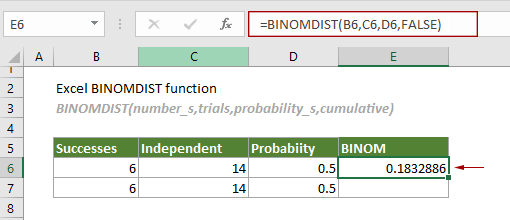Apply the BINOMDIST function to calculate the probability of at most 6 successes out of 14 trials..

Select a cell such as E7 in this case, copy or enter the formula below and press Enter to get the result.

=BINOMDIST(B7,C7,D7,TRUE)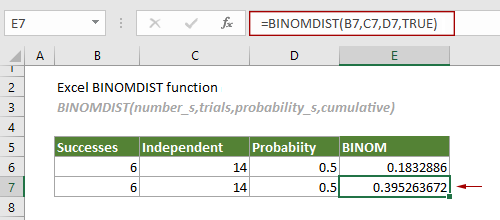#### Related Functions

Excel BINOM.DIST function
The BINOM.DIST function returns the individual term binominal distribution probability.

### The Best Office Productivity Tools

#### Kutools for Excel - Helps You To Stand Out From Crowd

 Popular Features: Find, Highlight or Identify Duplicates  |  Delete Blank Rows  |  Combine Columns or Cells without Losing Data  |  Round without Formula ... Super VLookup: Multiple Criteria  |  Multiple Value  |  Across Multi-Sheets  |  Fuzzy Lookup... Adv. Drop-down List: Easy Drop Down List  |  Dependent Drop Down List  |  Multi-select Drop Down List... Column Manager: Add a Specific Number of Columns  |  Move Columns  |  Toggle Visibility Status of Hidden Columns  |  Compare Columns to Select Same & Different Cells ... Featured Features: Grid Focus  |  Design View  |  Big Formula Bar  |  Workbook & Sheet Manager | Resource Library (Auto Text)  |  Date Picker  |  Combine Worksheets  |  Encrypt/Decrypt Cells  |  Send Emails by List  |  Super Filter  |  Special Filter (filter bold/italic/strikethrough...) ... Top 15 Toolsets:  12 Text Tools (Add Text, Remove Characters ...)  |  50+ Chart Types (Gantt Chart ...)  |  40+ Practical Formulas (Calculate age based on birthday ...)  |  19 Insertion Tools (Insert QR Code, Insert Picture from Path ...)  |  12 Conversion Tools (Numbers to Words, Currency Conversion ...)  |  7 Merge & Split Tools (Advanced Combine Rows, Split Excel Cells ...)  |  ... and more

Kutools for Excel Boasts Over 300 Features, Ensuring That What You Need is Just A Click Away...#### Office Tab - Enable Tabbed Reading and Editing in Microsoft Office (include Excel)

• One second to switch between dozens of open documents!
• Reduce hundreds of mouse clicks for you every day, say goodbye to mouse hand.
• Increases your productivity by 50% when viewing and editing multiple documents.
• Brings Efficient Tabs to Office (include Excel), Just Like Chrome, Edge and Firefox.